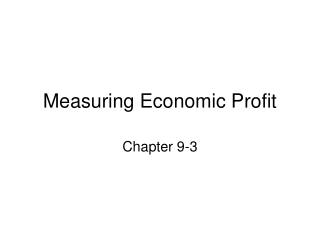DownloadDownload PresentationMeasuring Economic Profit

# Measuring Economic Profit

Télécharger la présentation## Measuring Economic Profit

- - - - - - - - - - - - - - - - - - - - - - - - - - - E N D - - - - - - - - - - - - - - - - - - - - - - - - - - -
##### Presentation Transcript

1. Measuring Economic Profit Chapter 9-3

2. Firms Maximize Profit • Profit is the difference between total revenue and total cost. Profit = total revenue – total cost

3. Firms Maximize Profit • Economists and accountants measure profit differently. • Accountants focus on explicit costs and revenue. • Economist focus on both explicit and implicit costs and revenue.

4. Firms Maximize Profit • For an economist, total cost is explicit payments to factors of production plus the opportunity cost of the factors provided by the owners of the firm.

5. Firms Maximize Profit • Economists define total revenue as the amount a firm receives for selling its good or service plus any increase in the value of the assets owned by firms.

6. Firms Maximize Profit • For economists: Economic profit = (explicit and implicit revenue) Minus (explicit and implicit cost)

7. Accounting Profit • The profit figure reported in annual reports and income statements is accounting profit: Accounting Profit = PQ – (cost of land) - (cost of labor) – (cost of capital)

8. Economic Profit • Accounting profit does not include the cost of ownership – called equity capital. • Economic profit includes all opportunity costs. Economic profit = accounting profit – cost of equity capital

9. Economic Profit • Economists refer to a firm that subtracts value, whose cost of equity capital is greater than its accounting profit, as having negative economic profit. • A firm that neither adds value nor subtracts it is a firm whose revenue is sufficient to pay the costs of inputs, but generates nothing in excess of this. This result is referred to as zero economic profit. • If a firm is returning more to its owners than the owners’ opportunity cost, the firm is said to be earning a positive economic profit.

10. Role of Economic Profit • Economic profit operates as a coordinating factor in the economy. • When a firm earns a positive economic profit, investors in the firm are earning better returns than they normally would with competing investments. • Other investors will want to invest in firm, too. As a result, resources will flow to where they earn more.